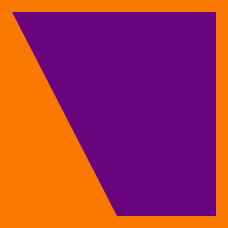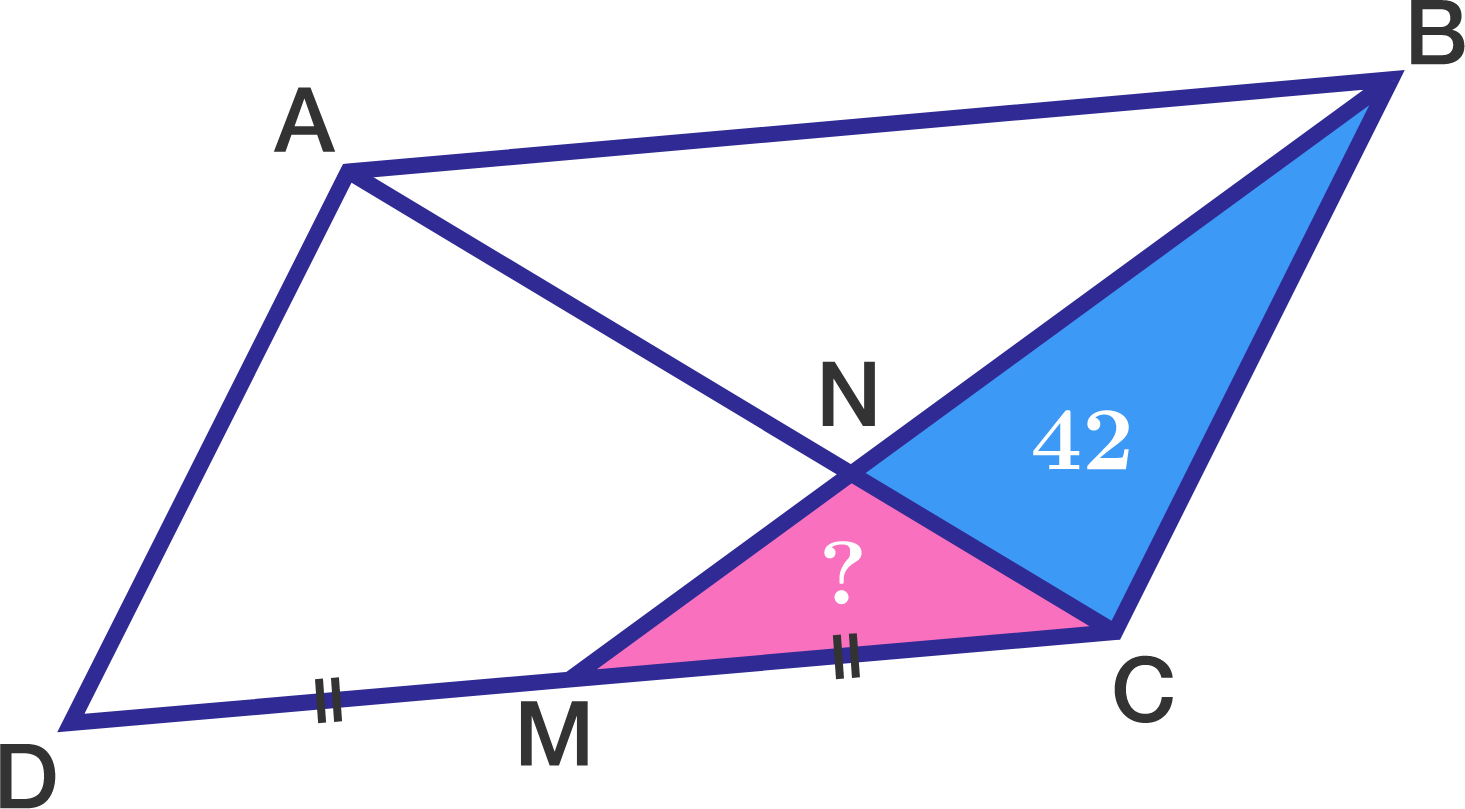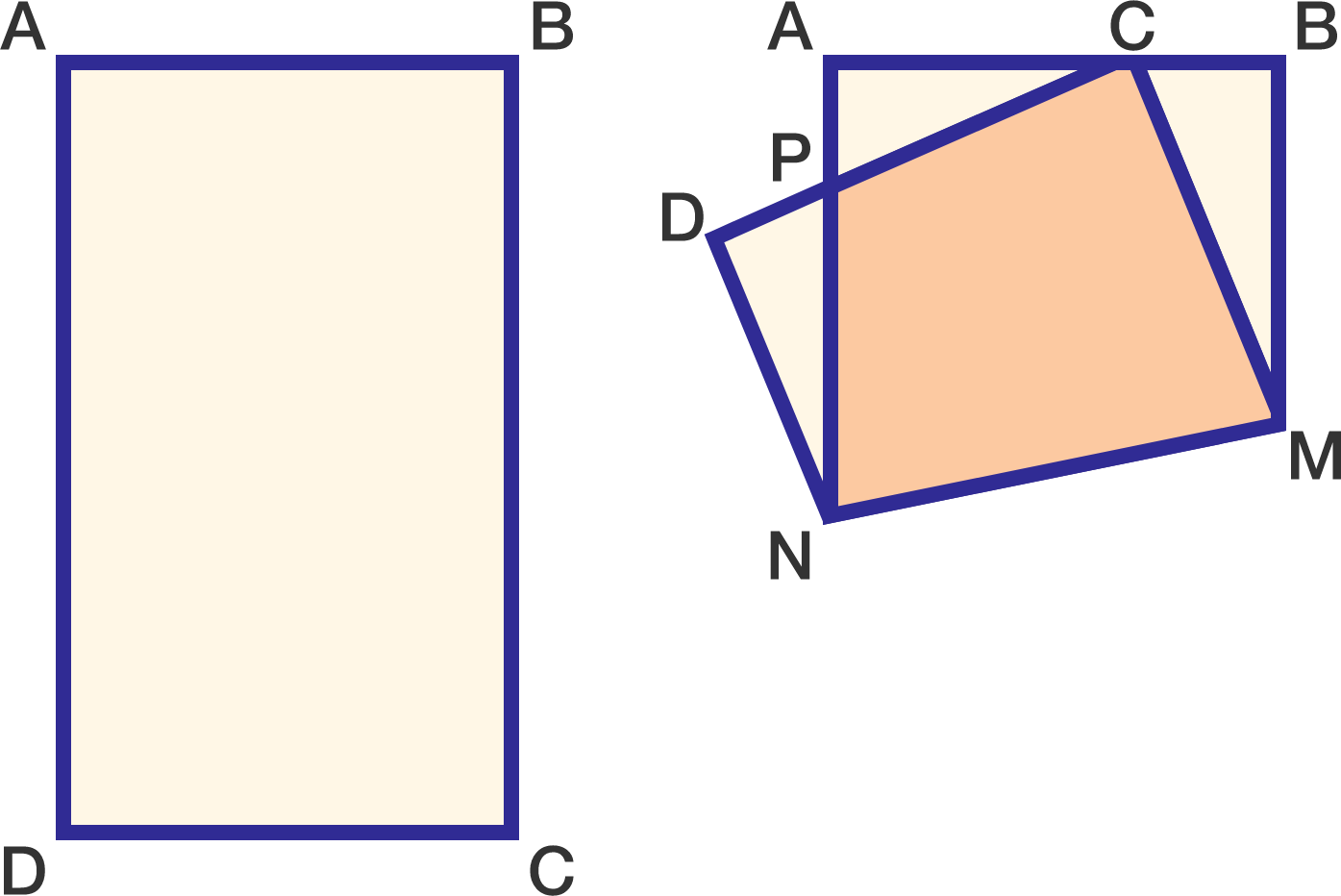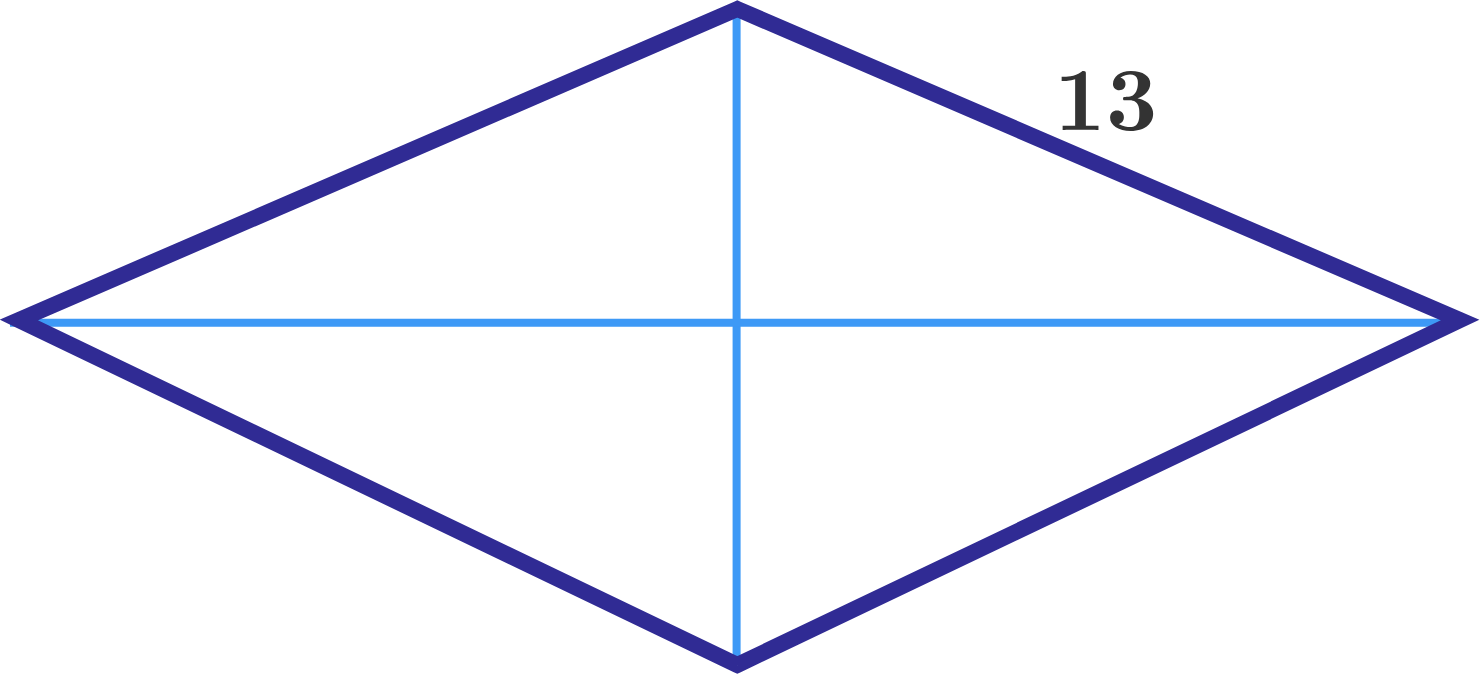Geometry

#### Challenge Quizzes

If the sides of a square are increased by 1%, then by what percent will the area of this square be increased?The above figure shows five congruent rectangles. A trapezoid is drawn in such a way that its vertices coincide with vertices of some of the rectangles. What percentage of the figure is shaded?In the diagram to the right, quadrilateral $ABCD$ is a parallelogram and $M$ is the midpoint of $\overline{DC}.$

If the area of $\triangle BNC$ is $42,$ what is the area of $\triangle MNC?$

In rectangle ABCD (below left), $AB=3$ and $BC=5$. The corner at C is folded up (below right) and lands on $\overline{AB}$ so that $AC=2$ and $CB=1$.The area of quadrilateral CPNM can be written as $\frac{m}{n}$, where m and n are positive, coprime integers. Find $m+n$.

What is the area of a rhombus of side 13 such that the sum of its two diagonals is 34?×